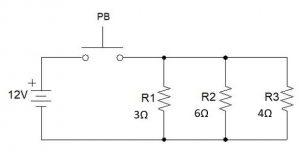# 1.4: Homework 1

$$\newcommand{\vecs}{\overset { \rightharpoonup} {\mathbf{#1}} }$$ $$\newcommand{\vecd}{\overset{-\!-\!\rightharpoonup}{\vphantom{a}\smash {#1}}}$$$$\newcommand{\id}{\mathrm{id}}$$ $$\newcommand{\Span}{\mathrm{span}}$$ $$\newcommand{\kernel}{\mathrm{null}\,}$$ $$\newcommand{\range}{\mathrm{range}\,}$$ $$\newcommand{\RealPart}{\mathrm{Re}}$$ $$\newcommand{\ImaginaryPart}{\mathrm{Im}}$$ $$\newcommand{\Argument}{\mathrm{Arg}}$$ $$\newcommand{\norm}{\| #1 \|}$$ $$\newcommand{\inner}{\langle #1, #2 \rangle}$$ $$\newcommand{\Span}{\mathrm{span}}$$ $$\newcommand{\id}{\mathrm{id}}$$ $$\newcommand{\Span}{\mathrm{span}}$$ $$\newcommand{\kernel}{\mathrm{null}\,}$$ $$\newcommand{\range}{\mathrm{range}\,}$$ $$\newcommand{\RealPart}{\mathrm{Re}}$$ $$\newcommand{\ImaginaryPart}{\mathrm{Im}}$$ $$\newcommand{\Argument}{\mathrm{Arg}}$$ $$\newcommand{\norm}{\| #1 \|}$$ $$\newcommand{\inner}{\langle #1, #2 \rangle}$$ $$\newcommand{\Span}{\mathrm{span}}$$$$\newcommand{\AA}{\unicode[.8,0]{x212B}}$$

Use the following schematic for questions 1, 2 and 3.1. Between which points would you expect to measure no voltage?
2. Between which points would you expect to measure source voltage?
3. The light bulb stops working. Using your meter, you read zero volts between points F and H and between E and J. You measure source voltage from I and B and from G and J. Where is the problem located?
4. Draw a ladder diagram of the following circuit:Wired Circuit
5. Using Ohm’s Law, fill in the missing values for the following circuit:Series Circuit
 R1 R2 R3 Total E 12V I R 3Ω 6Ω 4Ω
6. Using Ohm’s Law, fill in the missing values for the following circuit:Parallel circuit
 R1 R2 R3 Total E 12V I R 3Ω 6Ω 4Ω

7. Given the following schematic diagram with a control relay, siren and four switches, describe the behavior of this circuit in words.Speaker Circuit
8. How much current is in a 12V circuit that has 24kΩ of resistance?
9. How much voltage is present in a circuit with 30mA of current and 800Ω of resistance?
10. What resistance is present in a circuit with 120V and 80µA of current?
11. How would you write 2.4MΩ in scientific notation?
12. How would you write 2.4µA in scientific notation?
13. How might you write 3.45 x 108A?
14. How might you write 8.34 x 10-6Ω?
15. In the circuit below, draw in your meter to measure the following properties:
1. Resistance of R1
2. Voltage across R2
3. Current through R1, R2, and R3